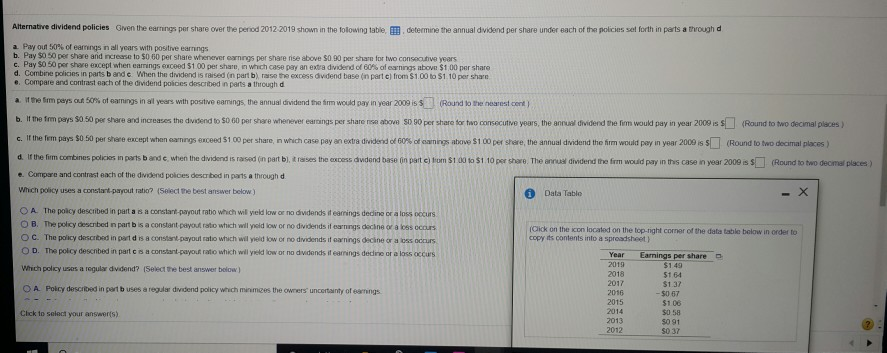# Alternative dividend policies Given the earnings per share over the period 2012 2019 shown in the...

###### Question:Alternative dividend policies Given the earnings per share over the period 2012 2019 shown in the to owing table determine the annual dividend por share under each of the policies set forth in parts a through d a Payout 50% of eamings in all years with positive earnings b. Pay $0.50 per share and increase to$0.50 per share whenever earnings per share rise above $0.90 per share for two consecutive years c. Pay 50 50 per share except when earnings cacoed$100 per share in which case pay an extra dividend of 60% of earnings above $100 per share d. Combine policies in parts and when the dividend isted in part b ase the excess dividend base in parte from$1.00 to $1.10 per share . Compare and contrast each of the dividend po ces described in parts a through d a t the firm pays out 50 gs in all years with postive cas e dividend the firm would win year 2009 iss R ound to the nearestent b. The firm pays SO 50 per share and increases the dividend to 1000 per share whenever e nds per share above 50 90 per share for two Goncutive years, the annual dividend them would pay in year 2009 is (Round to two decimal places c. If the fem pays$0.50 per share except when earnings exceed 5100 per share, in which case pay an extra dividend of 60% of wings atce $100 per shore, the annual dividend the firm would pay in year 2009 is 5 (Round to two decimal places) d. If the firm combines policies in parts and when the dividend is rased in part b), raises the excess dividend base in partium$100 to $1 10 per share. The annual dividend the firm would pay in this case in year 2009 is (Round to two decimal places) e. Compare and contrasteach of the dividend policies described in parts a through d Which policy uses a constant payout ratio? (Select the best answer below Data Table Click on the con located on the top right comer of the datatable below in order to copy its contents into a spreadsheet) OA. The policy described in part as a constant payout ratio which will yield low or no dividends it earnings dedine or a loss occurs OB. The policy descrbed in part bisa constant payout isto which will low or no dividends i ngedie cours OC. The policy described in pod is a constant payout ratio which will low or no dividends an d cus OD. The policy described in partes constant payout ratio which will low or no dividends fans de c o co Which policy uses a reguladdend? (Select the best answer below) Earnings per share 2018 O A Policy described in perib uses a regular dividend policy which minumees the owners uncertainty of e ngs 2016 2015$137 - $0.07$100 $0 58 SO 91$037 Click to select your answers) 2013 2012

#### Similar Solved Questions

##### The time needed to complete a final examination in a particular college course is normally distributed...
The time needed to complete a final examination in a particular college course is normally distributed with a mean of 77 minutes and a standard deviation of 12 minutes. Answer the following questions. a. What is the probability of completing the exam in one hour or less (to 4 decimals)? .0228 b. Wha...
##### Using nodal analysis in Figure 1, find the power that the 75 ohm resistor dissipates.
Using nodal analysis in Figure 1, find the power that the 75 ohm resistor dissipates....
##### Y=5/3(1-x)^2+cos^2(x+1) find dy/dx?
Y=5/3(1-x)^2+cos^2(x+1) find dy/dx?...
##### An economy consists of three workers: Larry, Moe and Curly. Each works 10 hours a day...
An economy consists of three workers: Larry, Moe and Curly. Each works 10 hours a day and can produce 2 services: mowing lawns and washing cars. In an hour Larry can either mow 1 lawn or wash 1 car; Moe can either mow 1 lawn or wash 2 cars; and Curly can either mow 2 lawns or wash 1 car. If all thre...
##### QUESTION 18 INSTRUCTIONS: Select the answer that best characterizes each a argument. Either you support the...
QUESTION 18 INSTRUCTIONS: Select the answer that best characterizes each a argument. Either you support the administration's policies or you don't deserve to be called a patriotic American. The choice should be obvious. a. No fallacy. b. False dichotomy. c. Accident Od. Suppressed evidence, ...